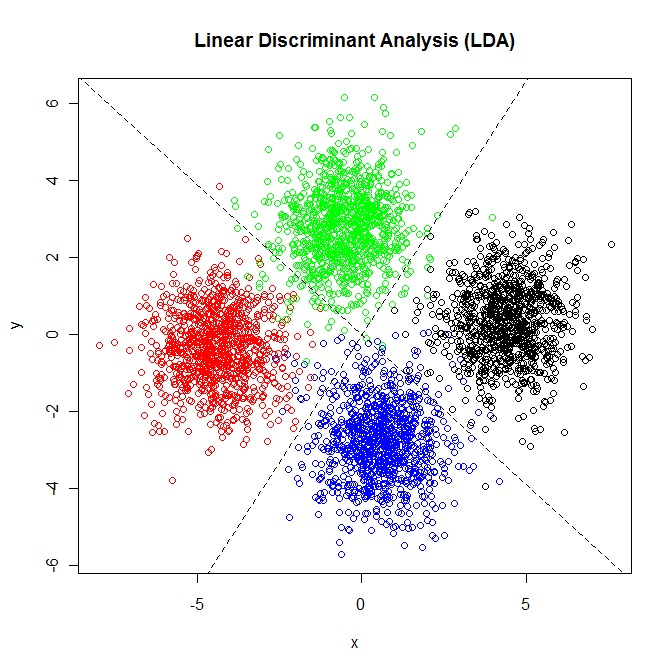# Statistical Modelling Services

Statistical modelling has two main purposes: prediction and explanation.

PredictionGiven a set of input values, what is the output?  Given a one unit change in a particular type of input, what is the effect on the output?

ExplanationHow do the variables relate to each other?  How strong is this relationship?  How much of the variation in the dependent variable is explained by the model?

There are many different statistical modelling techniques Statistical Consultants Ltd can provide, including (but not limited to) the following:

## Regression Models

Regression involves estimating the mathematical relationship between one variable called the response variable, and one or more explanatory variables.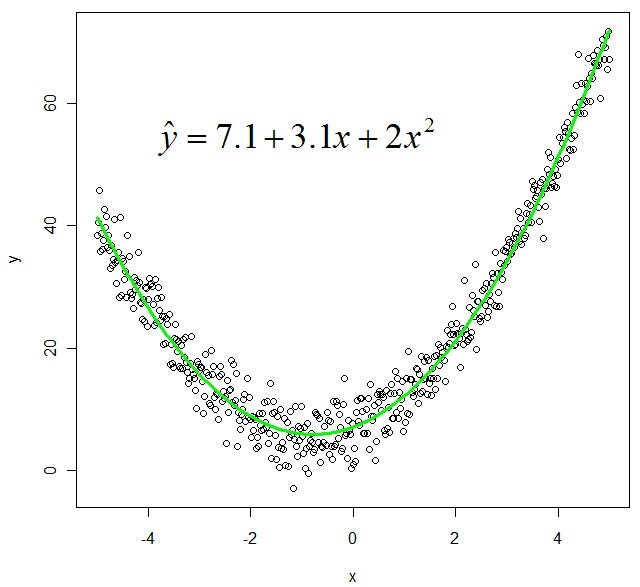Regression modelling can be used for forecasting time series data.## Nonparametric Models

Nonparametric models differ from parametric models (such as regression models), in that the model is data driven rather than structured with parameters to be estimated.

In the following plot, a smoother function was applied to the data: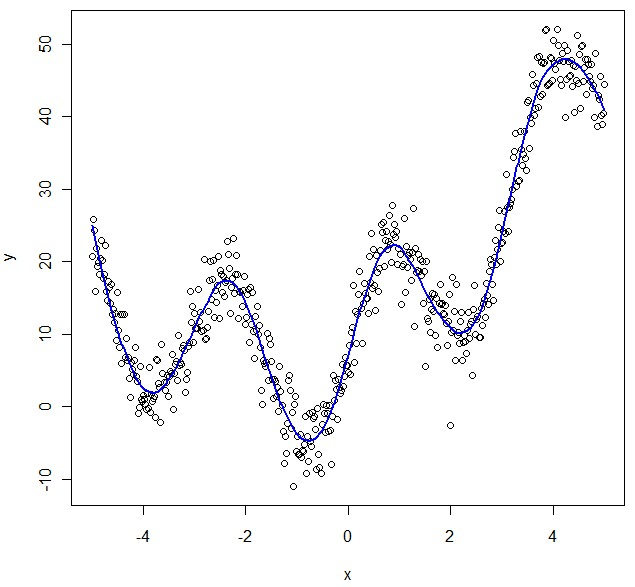## Semiparametric Models

Semiparametric models use a blend of parametric and nonparametric modelling techniques e.g. a regression model with a smoother component for greater flexibility.

## Bayesian Models

Bayesian modelling has become increasingly popular in recent years due to the rise of computer technology.  Bayesian models are based on probabilities rather than frequencies e.g. the probability of A, given B, C and D.  Bayesian models are based around Bayes’ Theorem:## Classification and Regression Trees

Classification and Regression Trees (CART) take a rather blunt approach, where a hierarchical structure is fitted to the data.  Classification trees are used for splitting categorical data.  Regression trees are used for splitting quantitative data.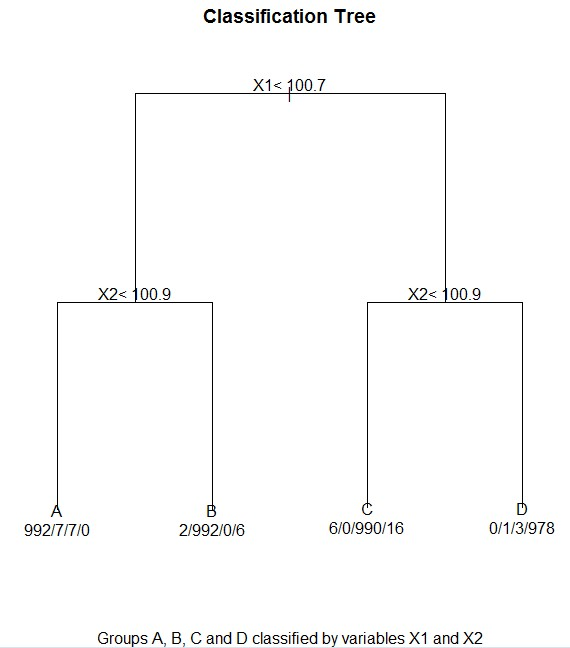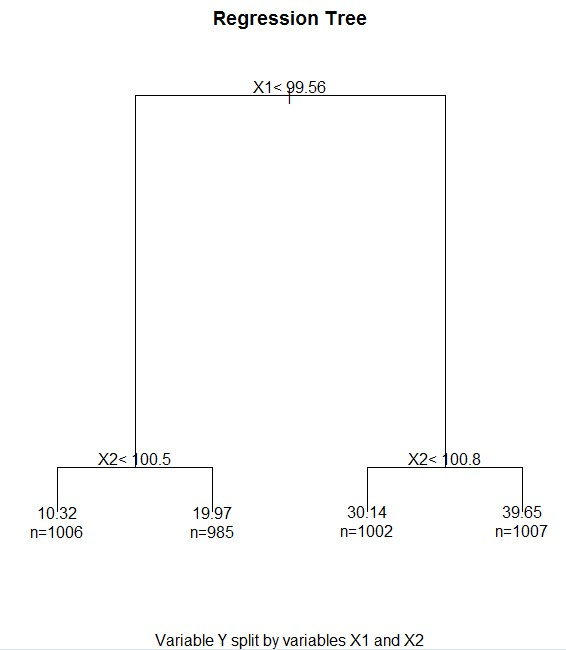## Discriminant Analysis

Discriminant analysis involves finding relationships which best separate the data into known groups.  When used for prediction, discriminant analysis models classify observations into groups.